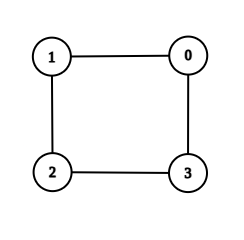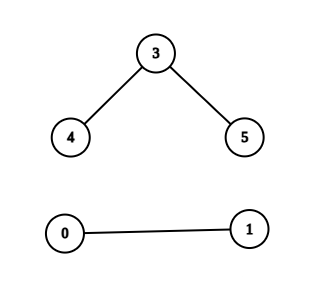New update is available. Click here to update.

# Get Path using BFS

Contributed by
Last Updated: 23 Feb, 2023
Easy0/40
Avg time to solve 25 mins
Success Rate 70 %Share7 upvotes

## Problem Statement

#### Find the path using BFS and print the first path that you encountered.

##### Note:
``````Vertices are numbered through 0 to V - 1.
``````
Detailed explanation ( Input/output format, Notes, Images )##### Constraints :
``````1 <= T <= 10
1 <= V <= 1000
1 <= E <= (V * (V - 1)) / 2
0 <= v1, v2 <= V-1

Time Limit: 1sec
``````
##### Sample Input 1 :
``````2
4 4
0 1
0 3
1 2
2 3
1 3
6 3
5 3
0 1
3 4
0 3
``````
##### Sample Output 1 :
``````true
false
``````
##### Explanation For Sample Output 1 :``````In the first test case, there are two paths from 1 to 3. I.e. 1 -> 2 -> 3 or 1 -> 0 -> 3. So you can print any one of them.
````````````In the second test case, there is no path from 0 to 3. Hence return an empty string.
``````
##### Sample Input 2 :
``````2
2 1
0 1
1 0
5 4
0 1
1 2
2 3
3 4
0 4
``````
##### Sample Output 2 :
``````true
true
``````AutocompleteConsole